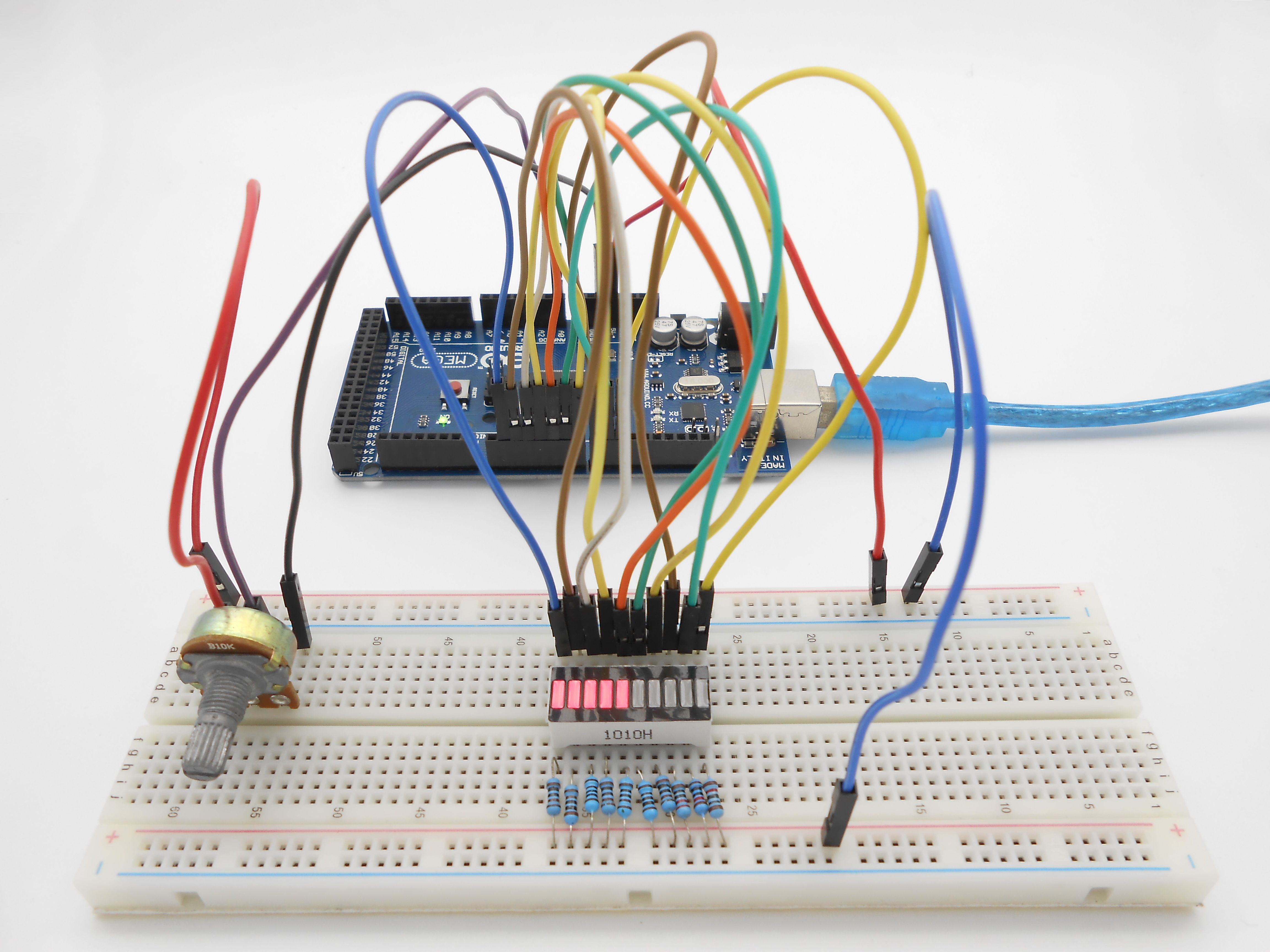No related product information!

# Lesson 7 LED bar graph display

### 1398

Overview

In this lesson, we will learn how to control a LED bar graph by programming the Arduino.

Requirement

- 1* Arduino MEGA 2560

- 1* USB Cable

- 1* 10KΩ Potentiometer

- 10* 220Ω Resistor

- 1* LED Bar Graph

- Several Jumper Wires

Principle

The bar graph - a series of LEDs in a line, such as you see on an audio display is a common hardware display for analog sensors. It's made up of a series of LEDs in a row, an analog input like a potentiometer, and a little code in between. You can buy multi-LED bar graph displays fairly cheaply. This tutorial demonstrates how to control a series of LEDs in a row, but can be applied to any series of digital outputs.

This tutorial borrows from the For Loop and Arrays tutorial as well as the Analog Input tutorial.

The sketch works like this: first you read the input. You map the input value to the output range, in this case ten LEDs. Then you set up a for loop to iterate over the outputs. If the output's number in the series is lower than the mapped input range, you turn it on. If not, you turn it off.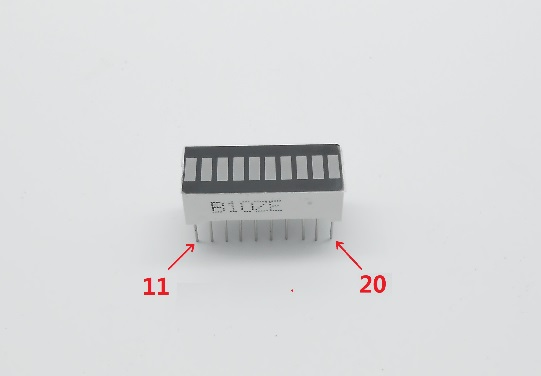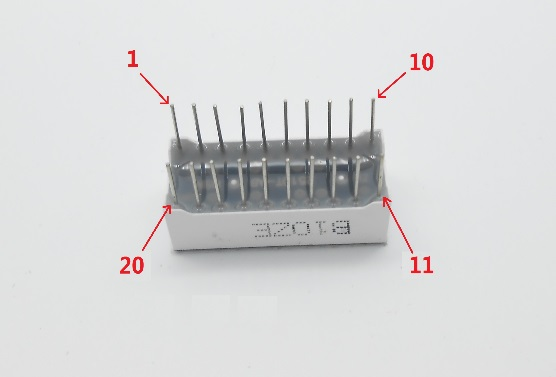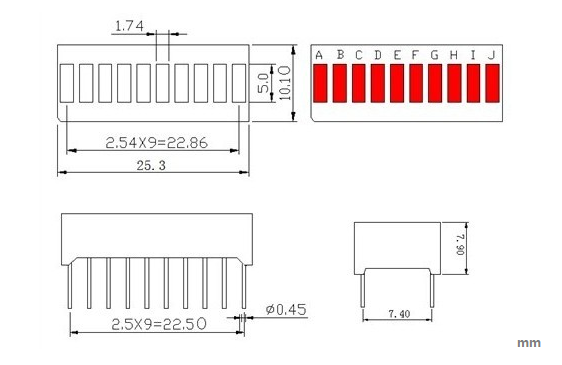The internal schematic diagram for the LED bar graph is shown below: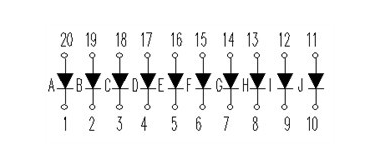A potentiometer, informally a pot, is a three-terminal resistor with a sliding or rotating contact that forms an adjustable voltage divider. If only two terminals are used, one end and the wiper, it acts as a variable resistor or rheostat.

Procedures

1. Build the circuit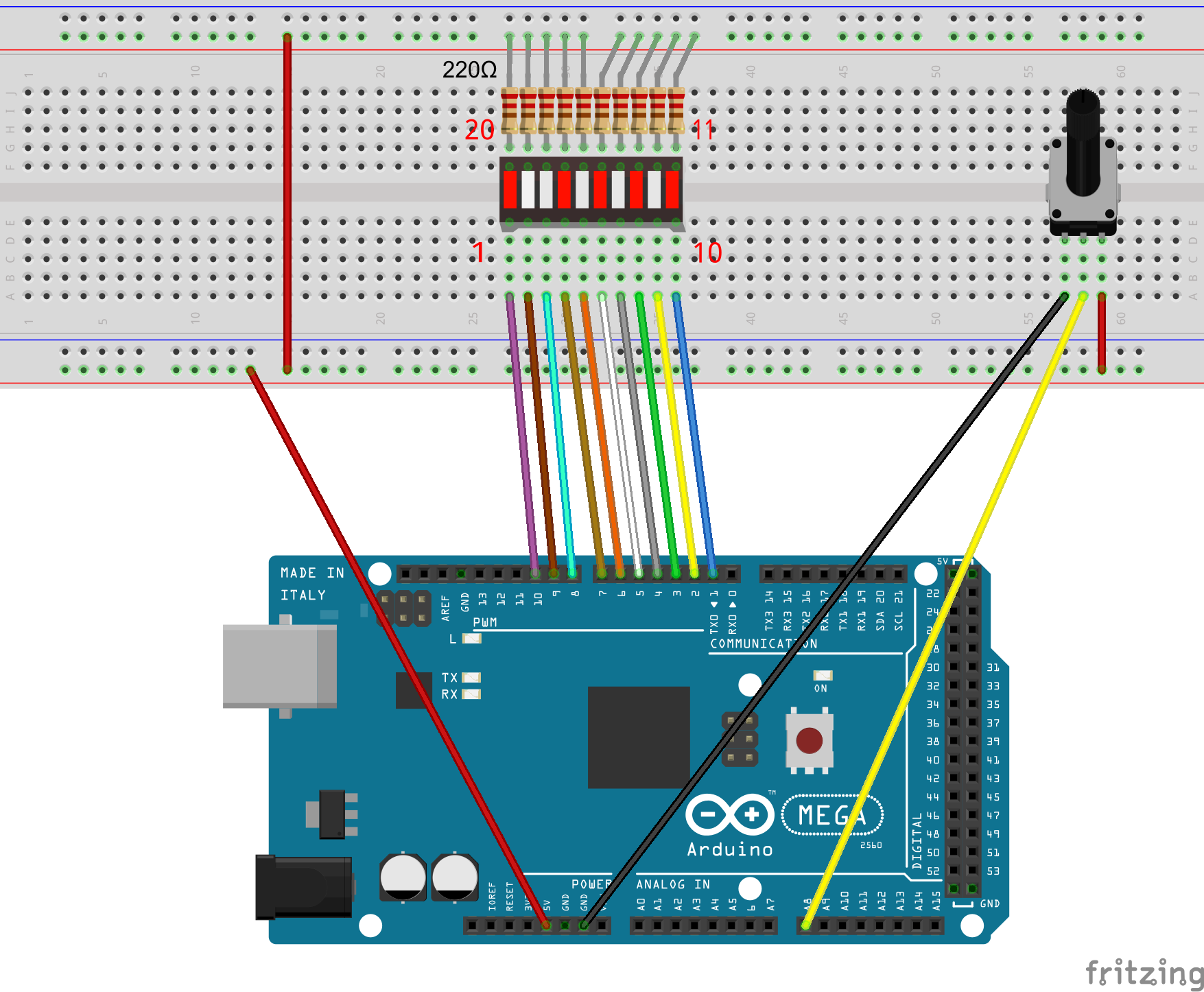2. Program_07_LEDBarGraph.ino

```/***********************************************************
File name: 07_LEDBarGraph.ino
Description: When you turn the potentiometer you will see
LED bar graph display changing.
Author: Tom
Date: 2015/12/27
***********************************************************/
int pin1 = 1;
int pin2 = 2;
int pin3 = 3;
int pin4 = 4;
int pin5 = 5;
int pin6 = 6;
int pin7 = 7;
int pin8 = 8;
int pin9 = 9;
int pin10 = 10;           //definition digital 10 pins as pin to control the LED

int potentiometerPin = 0; // potentiometer connected to analog pin 3

void setup()
{
pinMode(pin1,OUTPUT);    //Set the digital 1 port mode, OUTPUT: Output mode
pinMode(pin2,OUTPUT);    //Set the digital 2 port mode, OUTPUT: Output mode
pinMode(pin3,OUTPUT);    //Set the digital 3 port mode, OUTPUT: Output mode
pinMode(pin4,OUTPUT);    //Set the digital 4 port mode, OUTPUT: Output mode
pinMode(pin5,OUTPUT);    //Set the digital 5 port mode, OUTPUT: Output mode
pinMode(pin6,OUTPUT);    //Set the digital 6 port mode, OUTPUT: Output mode
pinMode(pin7,OUTPUT);    //Set the digital 7 port mode, OUTPUT: Output mode
pinMode(pin8,OUTPUT);    //Set the digital 8 port mode, OUTPUT: Output mode
pinMode(pin9,OUTPUT);    //Set the digital 9 port mode, OUTPUT: Output mode
pinMode(pin10,OUTPUT);   //Set the digital 10 port mode, OUTPUT: Output mode
}

void loop()
{
float a = analogRead(potentiometerPin);//Read the voltage photoresistance
a = map(a,0,1023,0,11);    //Photoresistor voltage value converted from 0-1023 to 0-11
for(int i=1;i<=a;i++){
digitalWrite(i,LOW);    //HIGH is set to about 5V PIN8
}
for(int j=10;j>a;j--){
digitalWrite(j,HIGH);   //HIGH is set to about 5V PIN8
}
delay(50);                 //delay 50ms
}```

3. Compile the program and upload to Arduino MEGA 2560 board

Now, when you turn the knob of the potentiometer, you will see that the number of LED in the LED bar graph will be changed.